## Chapter 8 Quadratic Equations R.D. Sharma Solutions for Class 10th Math Exercise 8.6

1. Determine the nature of the roots of the following quadratic equations:

(i) 2x2 - 3x + 5 = 0

Solution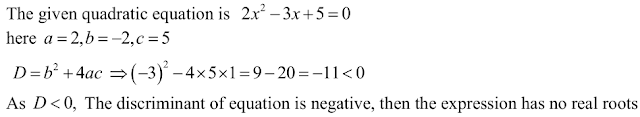(ii) 2x2 - 6x + 3 = 0

Solution

The given quadric equation is 2x2
Here , a = 2, b = -6 and c = 3
As we know that D = b2 – 4ac
Putting the value of a = 2, b = -6 and c = 3
= (-6)2 - 4 × 2 × 3
= 36 – 24
= 12
Since, D > 0
Therefore, root of the given are real and distinct .

(iii) 3/5 x2 - 2/3 x + 1 = 0

Solution(iv) 3x2 - 4√3 x + 4 = 0

Solution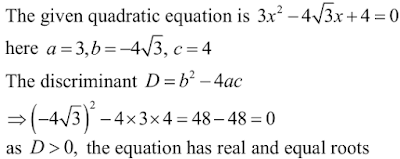(v) 3x2 - 2√6 x + 2 = 0

Solution2. Find the values of k for which the roots are real and equal in each of the following equation:

(i) kx2 + 4x + 1 = 0

Solution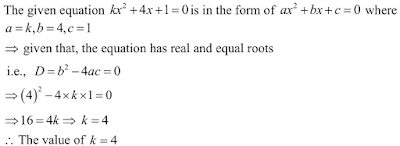(ii) kx2 - 2√5 x + 4 = 0

Solution(iii) 3x2 - 5x + 2k = 0

Solution(iv) 4x2 + kx + 9 = 0

Solution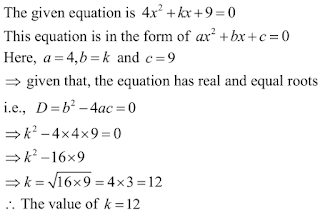(v) 2kx2 - 40 x + 25 = 0

Solution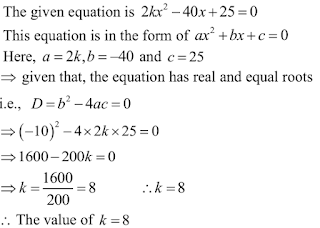(vi) 9x2 - 24x + k = 0

Solution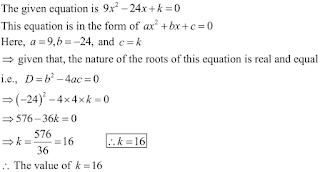(vii) 4x2 - 3kx + 1 = 0

Solution(viii) x2 - 2(5+2k)x + 3(7+10k) = 0

Solution(ix) (3k+1)x2 + 2(k+1) x + k = 0

Solution(x) kx2 + kx + 1 = -4x2 - x

Solution

The give question is kx2 + kx + 1 = -4x2 - x
⇒ kx2 + kx + 1+ 4x2 + x = 0
⇒ kx4x2 + kx + x + 1 = 0

x2 (k+4) + x(k + 1) + 1 = 0
Comparing with  ax2 + bx + c = 0
a = (k+4), b = (k+1), c = 1

Given that the nature of the roots of this equation are real and equal.
i.e. D = b2 - 4ac = 0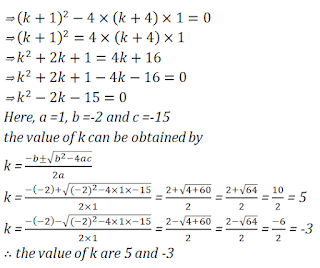(x) (k+1)x2 + 20 = (k+3)x + (k+8) = 0

Solution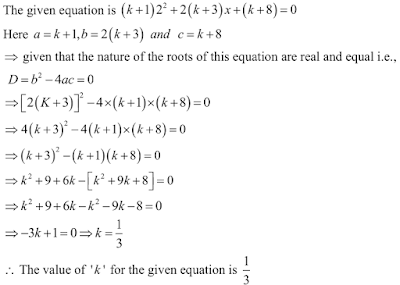(xii) x2 - 2kx + 7k - 12 = 0

Solution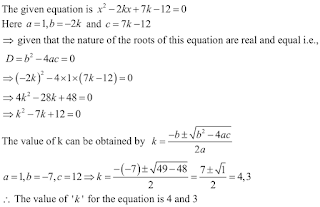(xiii) (k+1)x2 - 2(3k+1)x + 8k + 1 = 0

Solution(xiv) 5x2 - 4x + 2 + k(4k2 - 2x + 1) = 0

Solution(xv) (4-k)x2 + (2k + 4)x + (8k + 1) = 0

Solution(xvi) (2k+1)x2 + 2(k+3)x + (k+5) = 0

Solution(xvii) 4x2 - 2(k+1)x + (k+4) = 0

Solution3.In the following determine the set of values of k foe which the green quadratic equation has
real roots:
(i) 2x2 + 3x + k = 0

Solution(xvii) 2x2 + x + k = 0

Solution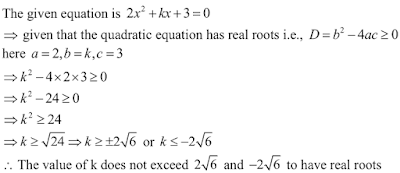(iii) 2x2 - 5x - k = 0

Solution(iv) kx2 + 6x + 1 = 0

Solution(v) 3x2 + 2x + k = 0

Solution4. Find the least positive value of k for which the equation have real and equal roots :

Solution

(i) x2 - 2(k+1)x + k2 = 0

Solution

(i) The given quadric equation is x2 -2 (k+1) x+k2 = 0, and roots are real and equal . Then find the value of k.
Here ,
a = 1, b = -2(k+1) and, c = k2
As we know that D = b2 – 4ac
Putting the value of a = 1, b = -2 (k+1) and, c =
= {-2(k+1)}2 -4 × 1 × k2
= {4(k2+2k+1)} – 4k2
= 4k2 + 8k + 4 – 4k2
= 8k + 4
The given equation will have real and equal roots, if D = 0
8k + 4 = 0
8k = -4
k = -4/8
= -1/2
Therefore, the value of k = -1/2

(ii) k2x2 - 2(2k-1)x + 4 = 0

Solution

The given quadric equation k2x2-2(2k-1)x+4 = 0 , and roots are real and equal
Then find the value of k.
Here,
a = k2, b = -2(2k-1) and , c = 4
As we know that D = b2 – 4ac
Putting the value of a = k2 , b = -2(2k-1) and, c = 4
= {-2(2k-1)}2 -4 × k2 × 4
= {4(4k2 – 4k + 1)} – 16k2
= 16k2 – 16k + 4 – 16k2
= -16k + 4
The given equation will have real and equal roots , if D = 0
-16k + 4 = 0
16k = 4
k =4/16
= 1/4
Therefore , the value of k = 1/4

(iii) (k+1)x2 - 2(k-1)x + 1 = 0

Solution

The given quadratic equation is (k+1)x2 – 2 (k-1)x+1 = 0, and roots are real and equal
Then find the value of k .
Here,
a = k+1, b = -2(k-1) and , c = 1
As we know that D = b2 – 4ac
Putting the value of a = k+1, b = -2(k-1) and , c = 1
= {-2(k-1)}2 – 4 × (k+1) × 1
= {4(k2 – 2k + 1)} – 4k – 4
= 4k2 – 8k + 4 – 4k – 4
= 4k2 – 12k = 0
The given equation will have real and equal roots, if  D = 0
4k2 – 12k + 0 = 0
4k2 – 12k = 0
Now factorizing of the above equation
4k(k-3) = 0
k(k-3) = 0
So, either
(k-3) = 0
k = 0 or
k = 3
Therefore, the value of k = 0, 3

5. Find the value of k for which the following equation have real roots

(i) 2x2 + kx + 3 = 0

Solution(ii) kx(x-2) +6 = 0

Solution(iii) x2 - 4kx + k = 0

Solution(iv) kx(x-2√5)+ 10 = 0

Solution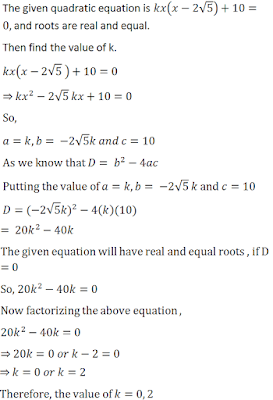(v) kx(x-3)+ 9 = 0

Solution(vi) 4x2 + kx + 3 = 0

Solution6. Find the values of k for which the given quadratic equation has real and distinct roots :

(i) kx2 + 2x + 1 = 0

Solution(ii) kx2 + 6x + 1 = 0

Solution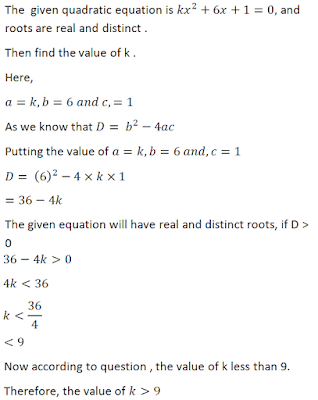7. For what value of k, (4-k)x2 + (2k+4)x+(8k+1) = 0, is a perfect square .

Solution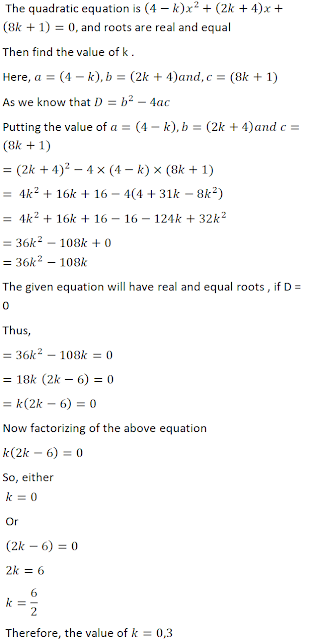8. Find the least positive value of k for which the equation x2+kx+4 = 0 has real roots .

Solution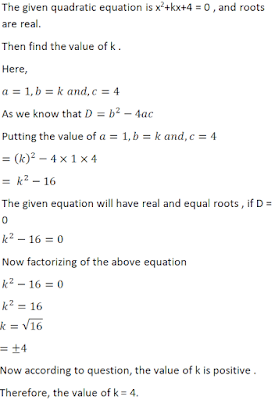9. Find the values of k for (3k + 1)x2 +2(k+1) x+1 = 0 has equal roots . Also, find the roots .

Solution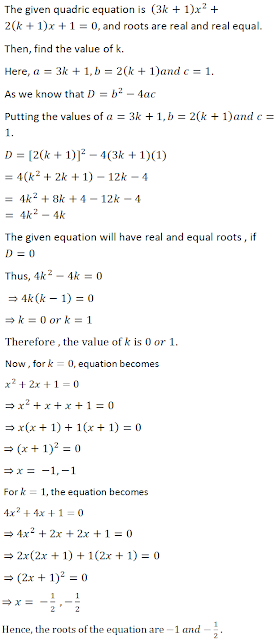10. Find the values of p for which the quadratic equation (2p+1)x2 – (7p+2)x+(7p-3)=0 has equal roots . Also find these roots .

Solution11. If -5 is a root of the quadratic equation 2x2 + px -15 = 0 and the quadratic equation p(x2+x)+k = 0 has equal roots , find the value of k.

Solution12. If 2 is a root of the quadratic equation 3x2 + px – 8 = 0 and the quadratic equation 4x2-2px + k = 0 has equal roots , find the value of k .

Solution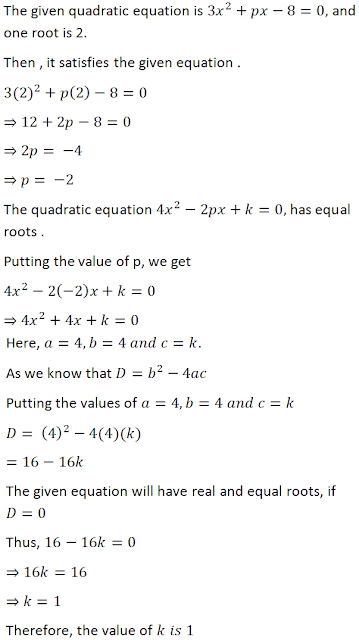13. If 1 is a root of the quadratic equation is 3x2 + ax – 2 = 0 and the quadratic equation a(x2 + 6x) – b = 0 has equal roots, find the value of b .

Solution

The given quadratic equation is3x2 + ax – 2 = 0 and one root is 1.
Then , it satisfies the given equation .
3(1)2 + a(1)-2=0
⇒ 3 + a – 2 = 0
⇒ 1+ a = 0
⇒ a = -1
The quadratic equation a(x2 + 6x) – b = 0 , has equal roots .
Putting the value of a , we get
-1(x2 + 6x) – b = 0
⇒ x2 + 6x + b = 0
Here . A = 1, B = 6 and C = b
D = (6)2 – 4(1) (b)
= 36 – 4b
The given equation will have real and equal roots , if   D = 0
Thus, 36 – 4b = 0
⇒ 4b = 36
⇒ b = 9
Therefore , the value of b is 9 .

14. Find the value of p for which the quadratic equation  (p+1)x2- 6(p+1) x + 3(p+9) = 0 , p ≠ -1 has equal roots . Hence, find the roots of the equation .

Solution

The given quadratic equation (p+1)x2- 6(p+1) x + 3(p+9) = 0 , has equal roots .
Here, a = p + 1, b = -6p -6 and c = 3p + 27.
As we know that D = b2 – 4ac
Putting the values of a = p + 1 , b = -6p – 6 and c = 3p +27.
D = [-6(p+1)]2 – 4(p+1)[3(p+9)]
= 36(p2 + 2p + 1) – 12(p2 + 10p + 9)
= 36p2 – 12p2 + 72p – 120p + 36 - 108
= 24p2 – 48 – 72
The given equation will have real and equal roots, if D = 0
Thus , 24p2 – 48p – 72 = 0
⇒ p2 – 2p – 3 = 0
⇒ p2 – 3p + p – 3 = 0
⇒ p(p -3) + 1 (p-3) = 0
⇒ (p+1)(p -3) = 0
⇒ p+1 = 0 or p – 3 = 0
⇒ p = -1 or p = 3
Therefore , the value of p is -1,3.
It is given that p ≠ 1, thus p = 3 only .
Now the equation becomes
4x2 -24x + 36 = 0
⇒ x2 – 6x + 9 = 0
⇒ x2 – 3x – 3x + 9 = 0
⇒ x(x-3)-3(x-3) = 0
⇒ (x-3)2 = 0
⇒ x = 3 , 3
Hence , the root of the equation is 3.

15. Determine the nature of the roots of the following quadratic equations :
(i) (x-2a) (x – 2b) = 4ab
(ii) 9a2b2x2-24abcdx + 16c2d2 = 0 , a ≠ 0, b ≠ 0
(iii) 2(a2+b2)x2 + 2(a+b)x+1 = 0
(iv) (b+c)x2 – (a+b+c) x + a = 0

Solution

(i) The given quadratic equation is (x-2a)(x-2b) = 4ab
⇒ x2 – 2(a+b)x + 4ab – 4ab = 0
⇒ x2 – 2(a+b) x = 0
Here, a = 1, b = -2(a+b) and, c = 0
As we know that D = b2 – 4ac
Putting the value of a = 1, b = -2(a+b) and , c = 0
= (-2(a+b))2-4×1×0
= 4(a2+2ab + b2) – 0
Since , D > 0
Therefore , root of the given equation are real and distinct .

(ii) The given quadratic equation is 9a2b2x2-24abcdx + 16c2d2 = 0
Here, a = 9a2b, b = -24abcd and, c = 16c2d2
As we know that D = b2 – 4ac
Putting the value of a = 9a2b2, b = -24abcd and, c = 16c2d2
= (24abcd)2 – 4 × 9a2b2×16c2d2
= (576a2b2c2d2) – 576a2b2c2d2
= 0
Since, D = 0
Therefore, root of the given equation are real and equal .

(iii) The given quadratic equation is 2(a2+b2)x2 + 2(a+b)x+1=0
Here , a = 2(a2+b2), b = 2(a+b) and, c = 1
As we know that D = b2 – 4ac
Putting the value of a = 2(a2+b2), b = 2(a+b) and, c = 1
= (2(a+b))2 – 4 × 2(a2 + b2) × 1
= (4a2 + 4b2 + 8ab) – 8a2 – 8b2
= 8ab – 4a2 – 4b2
Since, D < 0
Therefore , root of the given equation are not real.

(iv) The given quadratic equation is (b+c)x2 – (a+b+c)x+a = 0
Here, a = (b+c), b = -(a+b+c) and, c = a
As we know that D = b2 – 4ac
Putting the value of a = (b+c), b = -(a+b+c) and, c = a
= (-a(a+b+c))2 – 4 × (b+c) × a
= (a2 + b2 + c2 + 2ab + 2bc + 2ca) – 4ab – 4ca
= a2 + b2 + c2 – 2ab + 2bc – 2ca
Since, D > 0
Therefore, root of the given equation are real and unequal .

16.  Determine the set of values of k for which the following quadratic equations have real roots:

(i) x2-kx+9=0
(ii) 2x2 + kx + 2 = 0
(iii) 4x2 – 3kx + 1 = 0
(iv) 2x2 + kx – 4 = 0

Solution

(i) The given quadratic equation x2 – kx + 9 = 0, and roots are real
Then find the value of k .
Here, a= 1 , b = -k and, c = 9
As we know that D = b2 – 4ac
Putting the value of a = 1, b = -k and c = 9
= (-k)2 – 4 × 1 × 9
= k2 – 36
The given equation will have real roots , if D ≥ 0
k2 – 36 ≥ 0
k2 ≥ 36
k ≥ √36 or k ≤ - √36
k ≤ - 6 or k ≥ 6
Therefore, the value of k ≤ - 6 or k ≥ 6

(ii) The given quadratic equation is 2x2 + kx + 2 = 0, and roots are real.
Then find the value of k.
Here , a = 2 , b = k and , c = 2
As we know that D = b2 – 4ac
Putting the value of a = 2 , b = k and , c = 2
= (k)2 - 4×2×2
= k2 - 16
The given equation will have real roots , if D ≥ 0
k2 – 16 ≥ 0
k2 ≥ 16
k ≥ √16 or k ≤ - √16
k ≤ -4 or k ≥ 4
Therefore , the value of k ≤ -4 or k ≥ 4(iv) The given quadratic equation is 2x2 + kx – 4 = 0, and roots are real
Then find the value of k .
Here, a = 2, b = k and c , = -4
As we know that D = b2 – 4ac
Putting the value of a = 2 , b = k and c = -4
= (k)2 – 4 × 2 × (-4)
= k2 + 32
The given equation will have real roots , if D ≥ 0
k2 + 32 ≥ 0
since left hand side is always positive . So k ∈ R
Therefore , the value of k ∈ R

17. If the roots of the equation (b-c) x2 + (c-a)x+(a-b) = 0 are equal , then prove that 2b = a + c.

Solution18. If the roots of the equation (a2 + b2) x2 - 2(ac + bd) x + (c2 + d2) = 0 are equal ,  prove that a/b = c/d .

Solution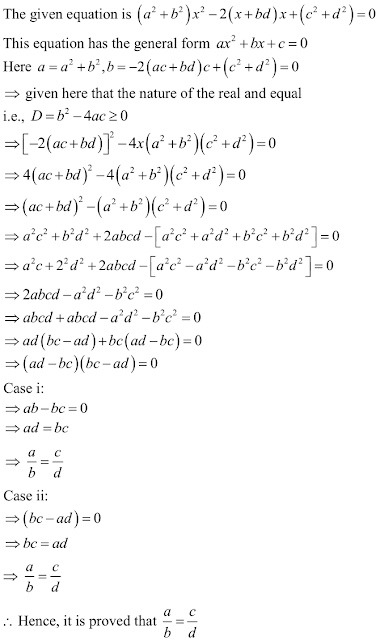19. If the roots of the equations ax2 + 2bx + c = 0 and bx2 – 2√acx + b = 0 are simultaneously real , then prove that b2 = ac .

Solution20. If p ,q are real and p ≠ q, then show that the roots of the equation (p-q)x2 +5(p+q) x – 2(p-q) = 0 are real unequal .

Solution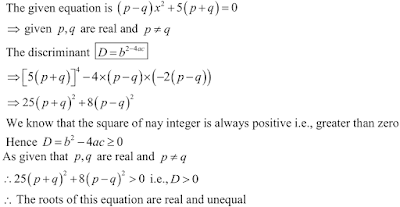21. If the roots of the equation (c2 – ab) x2 – 2(a2 – bc) x + b2 – ac = 0 are equal , prove that either a = 0 or a3 + b3 + c= 3abc.

Solution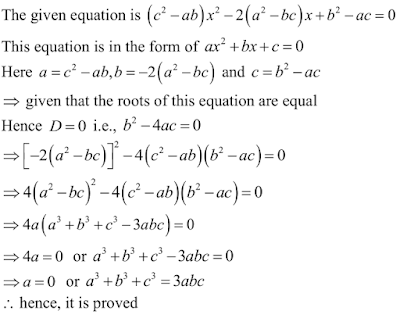22. Show that the equation 2(a2 + b2) x2 + 2(a+b) x + 1 = 0 has no real roots, when a ≠ b .

Solution23. Prove that both the roots of the equation (x-a) (x-b) + (x-b) (x-c ) + (x-c) (x-a) = 0 are real but they are equal only when a = b = c .

Sol.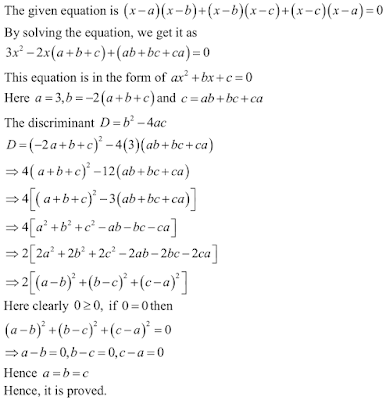24. If a,b,c are real numbers such that ac ≠ 0, then show that at least one of the equations ax2 + bx + c = 0 and - ax2 + bx + c = 0 has real roots .

Solution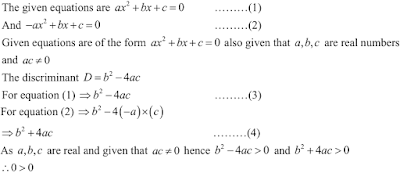25. If the equation (1+m2)x2 + 2mcx + (c2 – a2) = 0 has equal roots , prove that c2 = a2(1+m2).

Solution

The given equation (1+m2)x2 + 2mcx + (c2 – a2) = 0, has equal roots
Then prove that c2 = (1+m2).
Here,
a = (1+m2) , b = 2mc and , c = (c2 – a2)
As we know that D = b2 – 4ac
Putting the value of a = (1+m2) , b = 2mc and, c = (c2 – a2)
D = b2 – 4ac
= {2mc}2 – 4 × (1+m2) × (c2 – a2)
= 4 (m2c2) – 4(c2 a2 + m2c2 – m2a2)
= 4m2c2 – 4c2 + 4a2 – 4m2c2 + 4m2a2
= 4a2 + 4m2a2 – 4c2
The given equation will have real roots , if D = 0
4a2 + 4m2a2 – 4c2 = 0
4a2 + 4m2a2 = 4c2
4a2 (1+m2) = 4c2
a2(1+m2) = c2
Hence , c2 = a2 (1+m2)